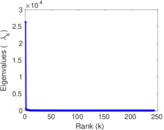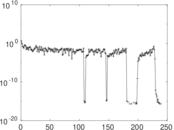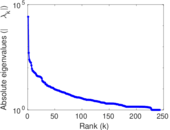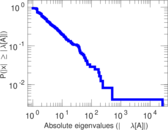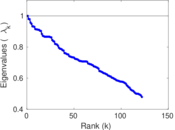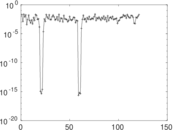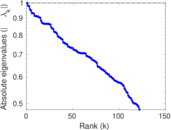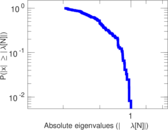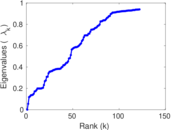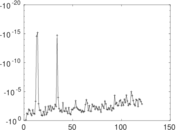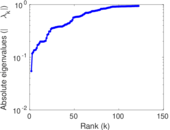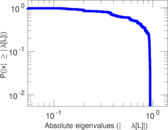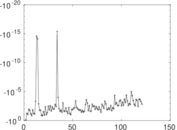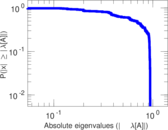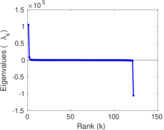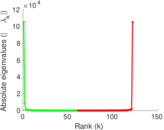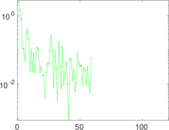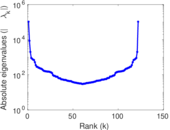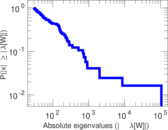# Wikiquote edits (hy)

This is the bipartite edit network of the Armenian Wikiquote. It contains users and pages from the Armenian Wikiquote, connected by edit events. Each edge represents an edit. The dataset includes the timestamp of each edit.

 Code `qhy` Internal name `edit-hywikiquote` Name Wikiquote edits (hy) Data source http://dumps.wikimedia.org/ AvailabilityDataset is available for download Consistency checkDataset passed all tests Category Authorship network Dataset timestamp 2017-10-20 Node meaning User, article Edge meaning Edit Network formatBipartite, undirected Edge typeUnweighted, multiple edges Temporal dataEdges are annotated with timestamps

## Statistics

 Size n = 8,674 Left size n1 = 380 Right size n2 = 8,294 Volume m = 70,203 Unique edge count m̿ = 21,596 Wedge count s = 19,060,527 Claw count z = 17,354,884,545 Cross count x = 13,556,557,571,721 Square count q = 8,287,896 4-Tour count T4 = 142,620,136 Maximum degree dmax = 31,005 Maximum left degree d1max = 31,005 Maximum right degree d2max = 26,311 Average degree d = 16.187 0 Average left degree d1 = 184.745 Average right degree d2 = 8.464 31 Fill p = 0.006 852 13 Average edge multiplicity m̃ = 3.250 74 Size of LCC N = 8,468 Diameter δ = 10 50-Percentile effective diameter δ0.5 = 3.156 53 90-Percentile effective diameter δ0.9 = 3.871 93 Median distance δM = 4 Mean distance δm = 3.247 22 Gini coefficient G = 0.876 750 Balanced inequality ratio P = 0.132 594 Left balanced inequality ratio P1 = 0.054 470 6 Right balanced inequality ratio P2 = 0.193 624 Relative edge distribution entropy Her = 0.728 360 Power law exponent γ = 2.499 37 Tail power law exponent γt = 2.721 00 Tail power law exponent with p γ3 = 2.721 00 p-value p = 0.000 00 Left tail power law exponent with p γ3,1 = 1.621 00 Left p-value p1 = 0.013 000 0 Right tail power law exponent with p γ3,2 = 8.071 00 Right p-value p2 = 0.911 000 Degree assortativity ρ = −0.295 568 Degree assortativity p-value pρ = 0.000 00 Spectral norm α = 26,310.2 Algebraic connectivity a = 0.054 423 0 Spectral separation |λ1[A] / λ2[A]| = 51.651 3 Controllability C = 7,945 Relative controllability Cr = 0.916 061

## Plots

### Fruchterman–Reingold graph drawing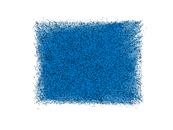### Degree distribution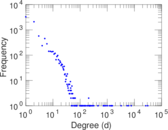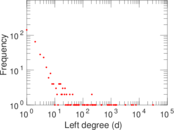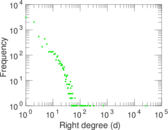### Cumulative degree distribution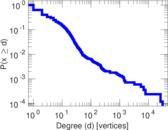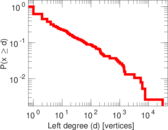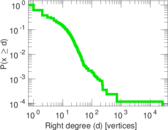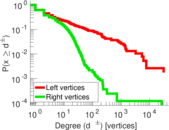### Lorenz curve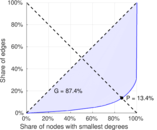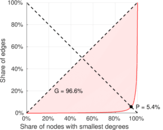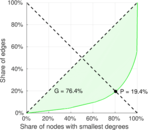### Spectral distribution of the adjacency matrix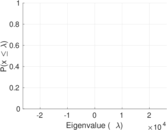### Spectral distribution of the normalized adjacency matrix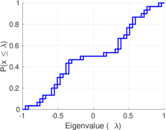### Spectral distribution of the Laplacian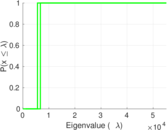### Spectral graph drawing based on the adjacency matrix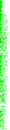### Spectral graph drawing based on the Laplacian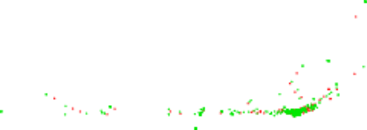### Spectral graph drawing based on the normalized adjacency matrix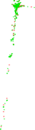### Degree assortativity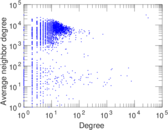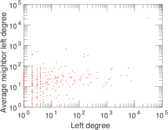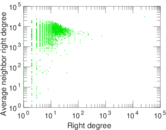### Zipf plot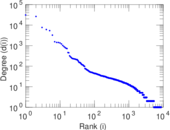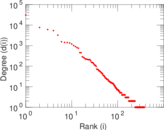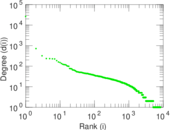### Hop distribution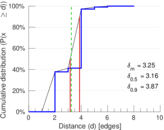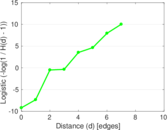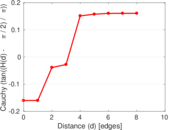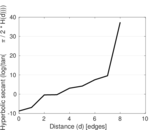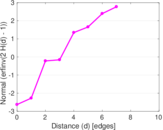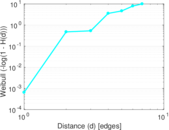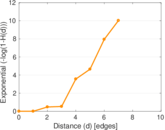### Double Laplacian graph drawing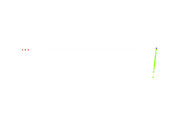### Delaunay graph drawing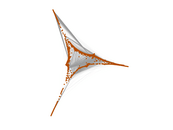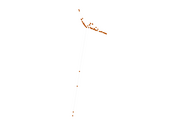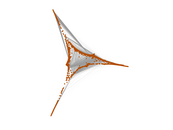### Edge weight/multiplicity distribution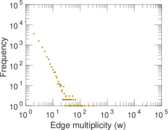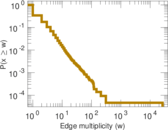### Temporal distribution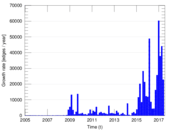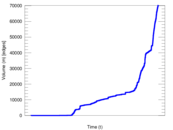### Temporal hop distribution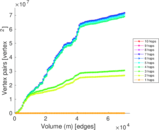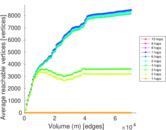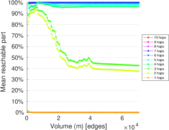### Diameter/density evolution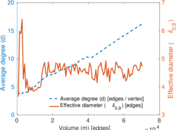### Matrix decompositions plots